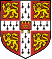The Cambridge Cluster Database

# Crystals for 60-, 256- and 320-atom binary Lennard-Jones mixtures

Binary Lennard-Jones mixtures are very popular in the simulation of glassy materials. The global minima presented here are for an 80-20 mixture, as first used by Kob and Anderson1, with parameters sigma(AA) = 1.0, sigma(AB) = 0.8, sigma(BB) = 0.88, epsilon(AA)=1.00, epsilon(AB) = 1.5 and epsilon(BB) = 0.5. The units of energy and length are epsilon(AA) and sigma(AA), respectively, and the number density is 1.2. We present the energies calculated using two different shifting techniques: Kob-Anderson (KA)1, which simply uses a constant to make the potential continuous at the cutoff; and Stoddard-Ford (SF)2 which uses a quadratic function so that the first derivatives are also continuous. We present results for a cutoff of 2.5 times the appropriate value of sigma for the both shifting techniques. For the SF technique we also include results for a cutoff of 1.842.

The systems presented here are not clusters, as they have periodic boundary conditions. The x,y, and z box lengths are contained in the first line of the xyz points files. The gifs for the 60- and 320-atom systems depict the 20- and 10- atom unit cells for these systems, respectively.

 N Energy (KA) Energy (SF, cutoff=2.5) Energy (SF, cutoff=1.842) Structure Coordinates 60 (cubic)3,4 -7.68 -7.08 -4.71 gif xyz-file 60 (orthorhombic)4 -7.77 -7.18 -4.85 gif xyz-file 2564 -7.87 -7.20 -4.74 gif xyz-file 3204 -7.99 -7.33 -4.90 gif xyz-file

All structures have been viewed and animated with XMAKEMOL.

1W. Kob and H. C. Andersen, Phys. Rev. Lett., 73, 1376 (1994)

2S. D. Stoddard and J. Ford, Phys. Rev. A., 8, 1504 (1973)

4T. F. Middleton, J. Hernández-Rojas, P. N. Mortenson and D.J.Wales, Phys. Rev. B., 64, 184201, 2001.